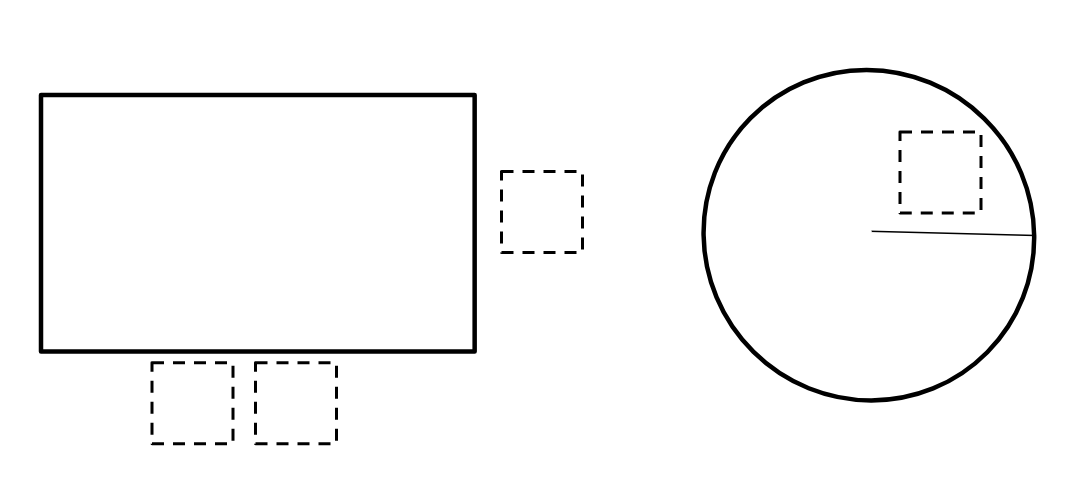Home > Grade 7 > Geometry > Perimeter & Circumference

# Perimeter & Circumference

Directions: Using the digits 1-6, at most one time each, fill in the boxes to create the largest and smallest combined perimeter/circumference for the rectangle and circle.### Hint

Which box number change has the greatest effect on the combined perimeter/circumference? Why?
What part of your calculations will be the same every time?

How many permutations are there of the numbers 1-6 in four positions?
Which permutations matter for the largest sum? Why?
Which permutations matter for the smallest sum? Why?

### Answer

greatest: (53 · 6) + (2π · 4) = 343.13… units

least: (24 · 1) + (2π · 3) = 42.85… units

Source: Christin Smith

## Converting a Fraction to a Decimal

Directions: Using the digits 1 to 9, at most one time each, place a digit …

### 7 comments

1.The solution given is actually for area of the rectangle and circumference of the circle

•Where do you put the numbers? Like which boxes? Thanks.

•You can choose to use any of the digits 1-6 in each of the boxes so that the rectangle has side lengths and the circle has a radius. You can only use each digit once.

2.Greatest: Rectangle with sides 63 and 4 and circle with radius 5. Answer: 165.4

•This is a solution but the one given in the answers is greater.

3.Least: Rectangle with sides 13 and 4 and circle with radius 2. Answer: 46.56

•Similarly with this solution Eric – it works but is not the smallest possible area.